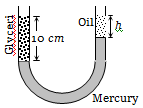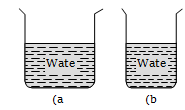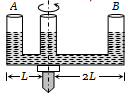A barometer tube reads 76 cm of mercury. If the tube is gradually inclined at an angle of 60o with vertical, keeping the open end immersed in the mercury reservoir, the length of the mercury column will be
(a) 152 cm                               (b) 76 cm
(c) 38 cm                                 (d) $38\sqrt{3}$ cm

Concept Questions :-

Pressure
High Yielding Test Series + Question Bank - NEET 2020

Difficulty Level:

A vertical U-tube of uniform inner cross section contains mercury in both sides of its arms. A glycerin (density = 1.3 g/${\mathrm{cm}}^{3}$) column of length 10 cm is introduced into one of its arms. Oil of density 0.8 gm/${\mathrm{cm}}^{3}$ is poured into the other arm until the upper surfaces of the oil and glycerin are in the same horizontal level. Find the length of the oil column. [Density of mercury = 13.6 g/${\mathrm{cm}}^{3}$](a) 10.4 cm               (b) 8.2 cm

(c) 7.2 cm                  (d) 9.6 cm

Concept Questions :-

Pressure
High Yielding Test Series + Question Bank - NEET 2020

Difficulty Level:

A triangular lamina of area A and height h is immersed in a liquid of density $\mathrm{\rho }$ in a vertical plane with its base on the surface of the liquid. The thrust on the lamina is

(a) $\frac{1}{2}\mathrm{A\rho gh}$                 (b) $\frac{1}{3}\mathrm{A\rho gh}$

(c) $\frac{1}{6}\mathrm{A\rho gh}$                  (d) $\frac{2}{3}\mathrm{A\rho gh}$

Concept Questions :-

Pressure
High Yielding Test Series + Question Bank - NEET 2020

Difficulty Level:

If two liquids of same masses but densities ${\mathrm{\rho }}_{1}$ and ${\mathrm{\rho }}_{2}$ respectively are mixed, then density of mixture is given by

(a) $\mathrm{\rho }=\frac{{\mathrm{\rho }}_{1}+{\mathrm{\rho }}_{2}}{2}$                             (b) $\mathrm{\rho }=\frac{{\mathrm{\rho }}_{1}+{\mathrm{\rho }}_{2}}{2{\mathrm{\rho }}_{1}{\mathrm{\rho }}_{2}}$

(c) $\mathrm{\rho }=\frac{2{\mathrm{\rho }}_{1}{\mathrm{\rho }}_{2}}{{\mathrm{\rho }}_{1}+{\mathrm{\rho }}_{2}}$                             (d) $\mathrm{\rho }=\frac{{\mathrm{\rho }}_{1}{\mathrm{\rho }}_{2}}{{\mathrm{\rho }}_{1}+{\mathrm{\rho }}_{2}}$

Concept Questions :-

Properties of fluids
High Yielding Test Series + Question Bank - NEET 2020

Difficulty Level:

If two liquids of same volume but different densities ${\mathrm{\rho }}_{1}$ and ${\mathrm{\rho }}_{2}$ are mixed, then density of mixture is given by

(a) $\mathrm{\rho }=\frac{{\mathrm{\rho }}_{1}+{\mathrm{\rho }}_{2}}{2}$                          (b) $\mathrm{\rho }=\frac{{\mathrm{\rho }}_{1}+{\mathrm{\rho }}_{2}}{2{\mathrm{\rho }}_{1}{\mathrm{\rho }}_{2}}$

(c) $\mathrm{\rho }=\frac{2{\mathrm{\rho }}_{1}{\mathrm{\rho }}_{2}}{{\mathrm{\rho }}_{1}+{\mathrm{\rho }}_{2}}$                           (d) $\mathrm{\rho }=\frac{{\mathrm{\rho }}_{1}{\mathrm{\rho }}_{2}}{{\mathrm{\rho }}_{1}+{\mathrm{\rho }}_{2}}$

High Yielding Test Series + Question Bank - NEET 2020

Difficulty Level:

The density $\mathrm{\rho }$ of water of bulk modulus B at a depth y in the ocean is related to the density at surface ${\mathrm{\rho }}_{0}$ by the relation

(a) $\mathrm{\rho }={\mathrm{\rho }}_{0}\left[1-\frac{{\mathrm{\rho }}_{0}\mathrm{gy}}{\mathrm{B}}\right]$                            (b) $\mathrm{\rho }={\mathrm{\rho }}_{0}\left[1+\frac{{\mathrm{\rho }}_{0}\mathrm{gy}}{\mathrm{B}}\right]$

(c) $\mathrm{\rho }={\mathrm{\rho }}_{0}\left[1+\frac{\mathrm{B}}{{\mathrm{\rho }}_{0}\mathrm{gy}}\right]$                             (d) $\mathrm{\rho }={\mathrm{\rho }}_{0}\left[1-\frac{\mathrm{B}}{{\mathrm{\rho }}_{0}\mathrm{gy}}\right]$

High Yielding Test Series + Question Bank - NEET 2020

Difficulty Level:

With rise in temperature, density of a given body changes according to one of the following relations

(a)                         (b)

(c)                                 (d)

Concept Questions :-

Properties of fluids
High Yielding Test Series + Question Bank - NEET 2020

Difficulty Level:

From the adjacent figure, the correct observation is(a) The pressure on the bottom of tank (a) is greater than at the bottom of (b).
(b) The pressure on the bottom of the tank (a) is smaller than at the bottom of (b)
(c) The pressure depend on the shape of the container
(d) The pressure on the bottom of (a) and (b) is the same

Concept Questions :-

Pressure
High Yielding Test Series + Question Bank - NEET 2020

Difficulty Level:

A given shaped glass tube having uniform cross section is filled with water and is mounted on a rotatable shaft as shown in figure. If the tube is rotated with a constant angular velocity $\mathrm{\omega }$ then(a) Water levels in both sections A and B go up
(b) Water level in Section A goes up and that in B comes down
(c) Water level in Section A comes down and that in B it goes up
(d) Water levels remains same in both sections

Concept Questions :-

Pressure

Difficulty Level:

An ice berg of density 900 Kg/${\mathrm{m}}^{3}$ is floating in water of density 1000 Kg/${\mathrm{m}}^{3}$. The percentage of volume of ice-cube outside the water is
(a) 20%                                       (b) 35%
(c) 10%                                       (d) 25%

Concept Questions :-

Archimedes principle